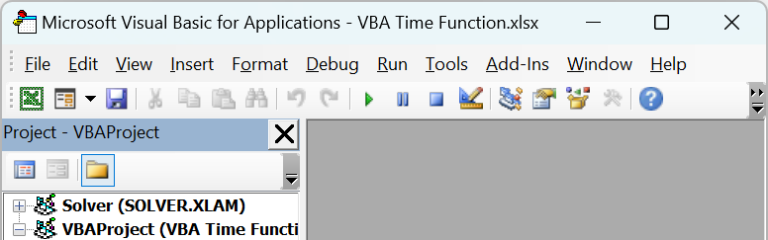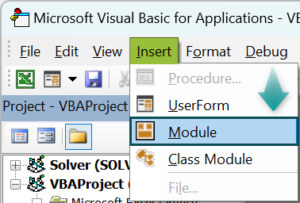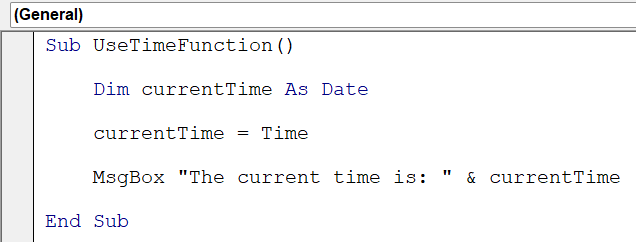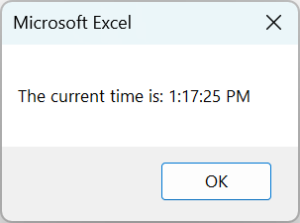# VBA Time FunctionArticle byExcelMojo Team## What is Excel VBA Time Function?

The Excel VBA Time Function is a built-in function that returns the current system time as a Variant data type. It allows developers to work with time-related data in Visual Basic for Applications (VBA) code.

The Time function retrieves the current time in hours, minutes, and seconds without the date information. The returned time is based on the computer’s system clock, making it useful for time-based calculations and recording timestamps in VBA macros. In the below example, we have a VBA macro named “CalculateElapsedTime” that calculates the time difference between two timestamps: “startTime” and “endTime.” It displays the elapsed time in hours, minutes, and seconds.

Suppose the “startTime” variable is set to July 22, 2023, 9:30:00 AM, and the “endTime” variable is set to July 22, 2023, 2:45:30 PM. When you run the “CalculateElapsedTime” macro, it will calculate the time difference between these timestamps and display the result as “The elapsed time is: 05:15:30” in the message box.

###### Key Takeaways
• The Excel VBA Time Function returns the current system time without the date information.
• Use the VBA Time function for time-based calculations and to record timestamps in VBA macros.
• The Format function can be used to format time values as per specific requirements.
• An alternative to the VBA Time function is DateAndTime.Now, which returns both date and time information.

### How to Use TIME Function in VBA?

To use the TIME function in VBA, follow these steps.

1. Open the Excel workbook and Press “Alt + F11” to open the Visual Basic for Applications (VBA) editor.2. In the VBA editor, click “Insert” in the menu and choose “Module” to insert a new module.3. You can write the VBA code using the VBA Time function in the module. For example:

Sub UseTimeFunction()
Dim currentTime As Date
currentTime = Time
MsgBox “The current time is: ” & currentTime
End Sub4. Press “F5” to run the macro and display the current time in a message box.### Examples

#### Example #1 – Calculate Time Difference

Suppose you have two-time values representing the start time and end time of an event. You want to calculate the time difference between these two times and display it in hours, minutes, and seconds’ format.

Using the VBA Time function and Format function, the script calculates the time difference and presents it in a user-friendly “hh:mm:ss” format.

• Step 1: In the new module, we will first create a subroutine “TimeDifferenceExample” and define the variables to store the start time, end time, and time difference. We will use the Date data type for all three variables.
• Step 2: Assign values to the “startTime” and “endTime” variables. For this example, we will hardcode the time values for demonstration purposes. You might obtain these values from user input or other data sources in a real-world scenario.
• Step 3: Calculate the time difference using the endTime minus startTime. The result will be stored in the timeDiff variable.
• Step 4: Display the time difference in a message box using the MsgBox function. We will use the Format function to show the time difference in the “hh:mm:ss” format.
• Step 5: Save the module and close the VBA. Now press Alt + F8 to open the Macro menu, select “TimeDifferenceExample,” and run the code.
• Step 6: Once you do the VBA Time function execution, the VBA code will display the time difference between the given start and end times.

Here is the full code:

Sub TimeDifferenceExample()
Dim startTime As Date, endTime As Date, timeDiff As Date
startTime = TimeValue(“10:30:00 AM”)
endTime = TimeValue(“2:45:30 PM”)
timeDiff = endTime – startTime
MsgBox “The time difference is: ” & Format(timeDiff, “hh:mm:ss”)
End Sub

#### Example #2

Suppose you have an Excel worksheet with a list of dates in column A. You must populate each date’s corresponding weekdays (Monday to Sunday) in column B.

By leveraging the VBA Time and Format function, the script extracts the weekdays from the dates in column A and populates them in column B. This automated approach eliminates the need for manual entry, saving time and ensuring accurate weekday data.

• Step 1: In the new module, we create a subroutine, “PopulateWeekdays,” and set up the worksheet where the data is located. Here, we also define variables for the worksheet, the last row with data in column A, the current date, and the weekday value.
• Step 2: Next, we set up a loop to iterate through the dates in column A, starting from the second row (as the first row contains headers). The loop will continue until the last row with data in column A is reached.
• Step 3: In this step, we will get the Current Date and Calculate Weekday. Inside the loop, we retrieve the current date from the current row in column A and store it in the “currentDate” variable.

Then, we use the VBA Time and Format functions to calculate the weekday value and store it in the “weekdayValue” variable.

• Step 4: We write the code to populate Weekday in Column B.
• Step 5: Next, we close the loop with the “Next” statement.
• Step 6: Save the module and close the VBA. Now press Alt + F8 to open the Macro menu and select “PopulateWeekdays” and run the code.
• Step 7: Once you run the code, the VBA Time Function will automatically populate the weekdays in the B column against each date.

Here is full code:

Sub PopulateWeekdays()
Dim ws As Worksheet
Set ws = ThisWorkbook.Sheets(“Sheet1”) ‘ Change “Sheet1” to your sheet name
Dim lastRow As Long
lastRow = ws.Cells(ws.Rows.Count, “A”).End(xlUp).Row
Dim currentDate As Date
Dim weekdayValue As String
For i = 2 To lastRow
currentDate = ws.Cells(i, “A”).Value
weekdayValue = Format(currentDate, “dddd”)
ws.Cells(i, “B”).Value = weekdayValue
Next i
End Sub

### Alternate of Now() Function

An alternative to the Now() function in VBA is the DateTime VBA data type combined with the TimeValue function. The DateTime data type stores both date and time information, while the TimeValue function extracts the time portion from a given date and returns it as a Variant data type.

Example:

Sub AlternativeNowFunction()
Dim currentTime As Variant
currentTime = TimeValue(FormatDateTime(Now, vbShortTime))
MsgBox “The current time is: ” & currentTime
End Sub

In this VBA code, we utilize the “FormatDateTime” function to obtain the current date and time using “Now” and then format it to display only the time portion in a short time format (hh:mm AM/PM).

The “TimeValue” function extracts the time part from the formatted date-time string, and we store it in the “currentTime” variable.

The MsgBox function displays the current time.

### Important Things to Note

• The VBA Time function retrieves the current system time based on the computer’s system clock.
• There are no VBA Time functions milliseconds to directly get the current time with milliseconds; it returns the time with precision up to seconds.
• When working with time-related calculations, ensure that the time format is in the correct 24-hour or 12-hour format, depending on your requirements.
• To execute the VBA Time Function format, you can use the Format function with appropriate format codes (e.g., “hh:mm:ss” for hours, minutes, and seconds).

1. How to get system time in VBA?

In VBA, you can get the system time using the “TimeValue(Now)” function, which extracts the time portion from the current date and time obtained with Now. This method returns the current system time as a Variant data type.

Alternatively, you can use the Time data type along with the “TimeSerial” function to directly represent the system time without the date component. The “TimeSerial” function takes the hour, minute, and second values as arguments and constructs a Time data type value.

2. How do I autofill time in Excel?

To autofill time in Excel, follow these steps:

1) Enter the starting time in the first cell.
2) In the next cell, enter the formula “=A1+TIME(hours, minutes, seconds)”, where “A1” is the reference to the starting time cell, and “hours,” “minutes,” and “seconds” represent the time duration you want to add.
3) Drag the fill handle of the second cell down to autofill the time formula to other cells.

3. How do I convert time to number in Excel VBA?

You can use the “TimeValue” function to convert time to a number. It converts a valid time expression (in string or date format) into a decimal number representing the time value. The resulting decimal number represents the fraction of a 24-hour day.

4. How to extract time from date in Excel VBA?

To extract the time from a date, you can use the “TimeValue” function, which directly extracts the time component from the date and returns it as a Date data type.

Alternatively, you can use the Hour, Minute, and Second functions to individually extract the hour, minute, and second components of the date and then combine them to display the extracted time in your desired format.